### Introduction

To make KLT tracking more robust with respect those changes due to illumination, we modify the KLT warping model to:

$$I(x) = e^α I(x + b) + β$$

where $α$ and $β$ are two additional scalar coefficients accounting for changes in image contrast and image brightness respectively. However, for fear of expanding the size of the Jacobian iteration matrix (4×4 instead of 2×2), an image normalization step is adopted before updating the set of tracking coefficients $b$. This normalization step consists of scaling (by $λ$) and translating (by $δ$) the current warped image $J$ so that the reference warped image $I$ and the current warped image $J$ have same mean brightness and variance.

\begin{aligned} λ&= std(I) / std(J) \newline δ&= mean(I) - λ mean(J) \newline J&= λJ + δ\end{aligned}

### Evaluation

The figure below shows the tracking results where the the blue circles represent the detected keypoints by goodFeaturesToTrack, yellow circles represent the tracked keypoints by KLT and red circles represent the tracked keypoints after removing the outliers by findHomography or findFundamentalMat (Blue circles $⊇$ Yellow circles $⊇$ Red circels).

We define the tracking rate = number of tracked keypoints / number of detected keypoints.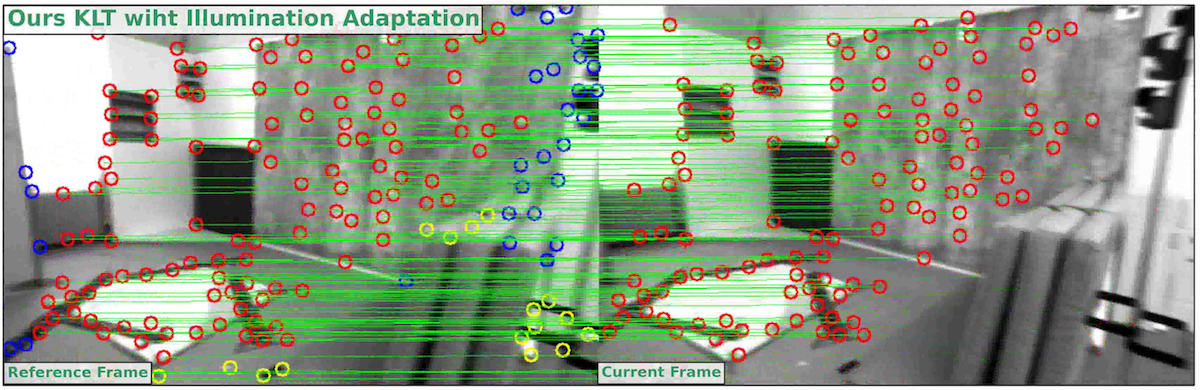#### Static Verification

The experiments below verify that our method extremely enhance the KLT tracking performance in spite of the illumination changes. Noteworthily, although original KLT has higher tracking rate without outlier removal in some experiments (Test2, Test5), it has a lower tracking rate after outlier removing, which means the result of original KLT contains numbers of false matching pairs. The false positive rate proves this.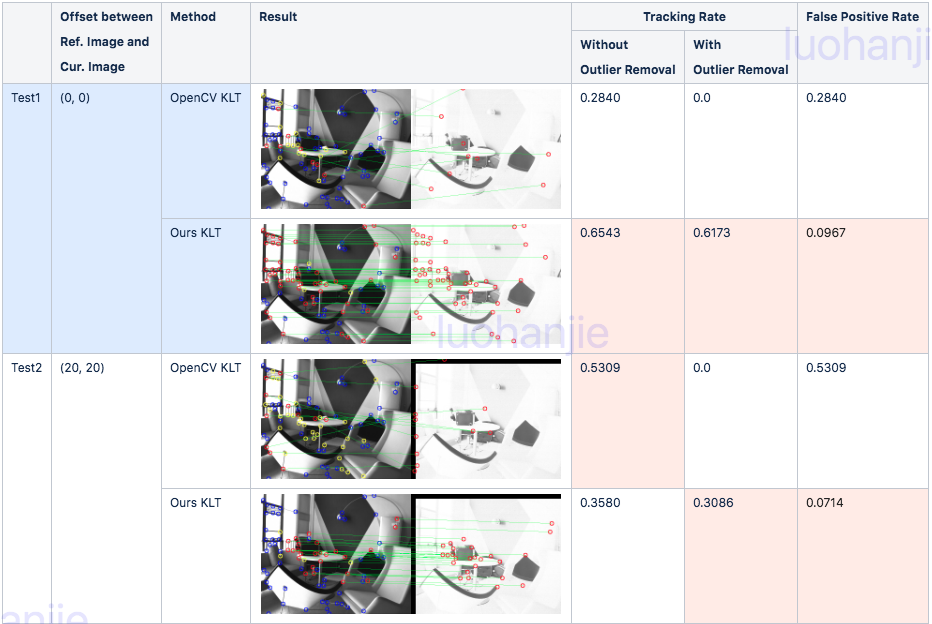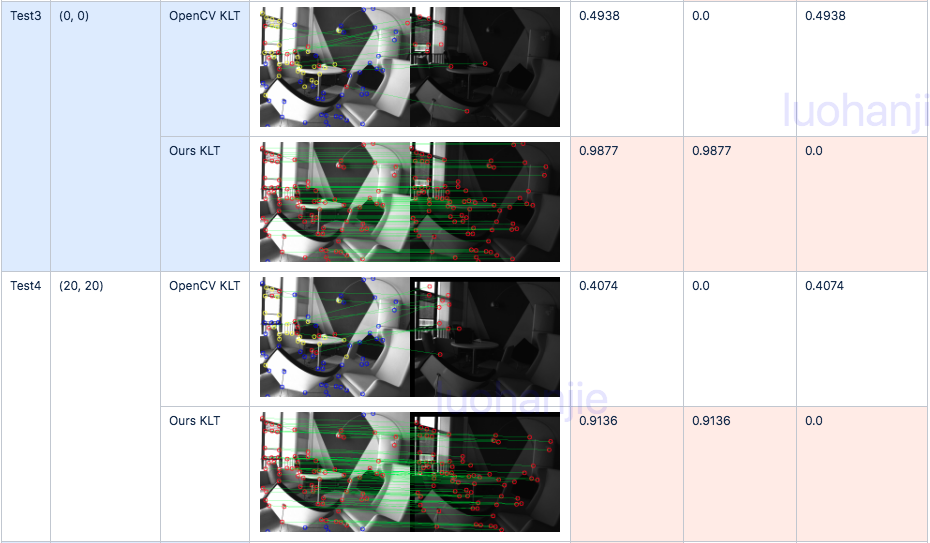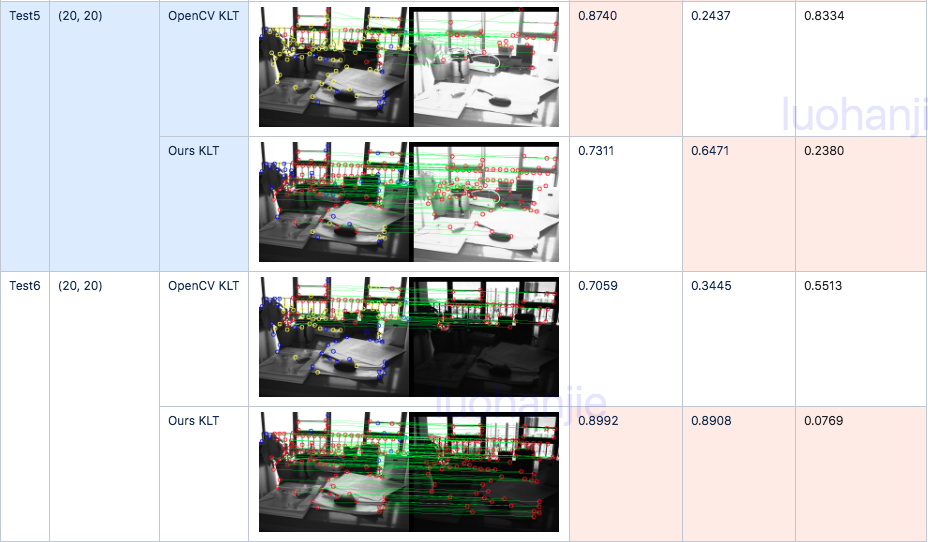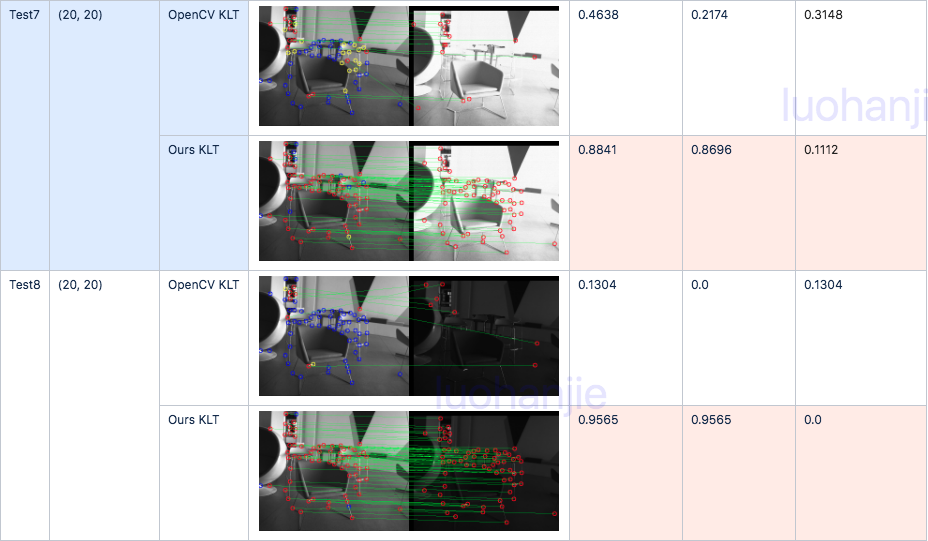#### Dynamic Verification

##### Evaluation Part 1

The new KLT is evaluated in the EuRoC V1_03_difficult Dataset, which is the most challenging dataset with aggressive motion and great illumination change. We take the current image as reference frame and the next image as the current frame.

###### Result##### Evaluation Part 2

A more challenging experiment, which takes the current image as reference frame and the next third image as the current frame, will be conducted.

###### Result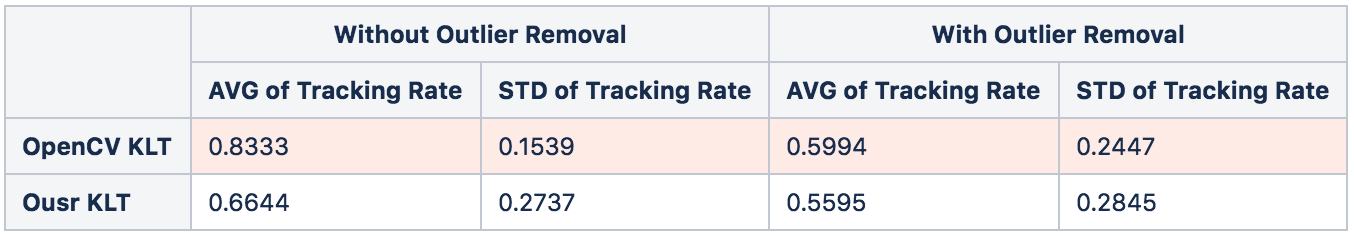It manifests the original KLT significantly outperforms our method. One probable reason is that the initial tracking position is too far to the tracked point in this experiment, convergence problem could arise for a more complex warping model. However, there are more outliers using the OpenCV KLT while the false positive rate is low using ours KLT.

1. Bouguet, Jean-Yves. "Pyramidal implementation of the affine lucas kanade feature tracker description of the algorithm." Intel Corporation 5.1-10 (2001): 4.

2. https://projects.asl.ethz.ch/datasets/doku.php?id=kmavvisualinertialdatasets